# Gamma & Log Gamma Function Calculator

Gamma Function Calculator Γ(Z)
Z =

Enter positive value for Z. Form accepts both decimals and fractions.

The gamma function, denoted by Γ, was developed to extend the factorial function over non-integers. If n is a non-negative integer, then the classical definition of n! is

n! = n⋅(n-1)⋅(n-2)⋅⋅⋅3⋅2⋅1, with 0! = 1

As it turns out, the factorial function can also be defined by the equation

n! = o xne-x dx.

This integral is defined for non-integer values of n, even complex values of n, thus providing a way to extend the factorial function over the real and complex numbers. The formal definition of the gamma function is

Γ(z) = o xz-1e-x dx.

For any positive integer z, Γ(z) = (z-1)!. The gamma function is important in many branches of mathematics, especially probability, statistics, and number theory. There are many equivalent representations of the gamma function as well as some nice functional relations. In general, one cannot compute the exact value of Γ(z) except when z is an integer or half-integer. However, you can use the calculator to generate accurate values for any positive input of z.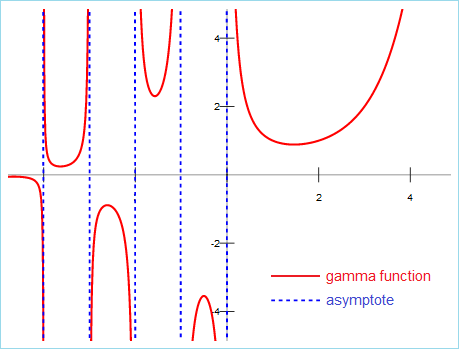## Other Representations of the Gamma Function

The integral definition is not the most convenient form of the gamma function since, in general, the integral cannot be evaluated in terms of elementary functions. However, there are more convenient infinite product representations of Γ(z):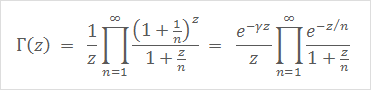Some functional relations allow you to evaluate Γ(z) in terms of other z values: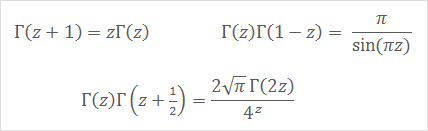## Special Values

If z is a negative integer or 0, the gamma function is undefined. When z is a positive integer, Γ(z) = (z-1)!. The value of Γ(0.5) can be found by evaluating the integral o x-0.5e-x dx and making the substitution x = y². This means Γ(0.5) = 2o e-y² dy. Fortunately, the value of this integral is known!

Γ(0.5) = 2o e-y² dy = √π

With this key value and the functional relations above, you can calculate the gamma function for all the half-integers. The general formulas and a few values are shown in the figure below: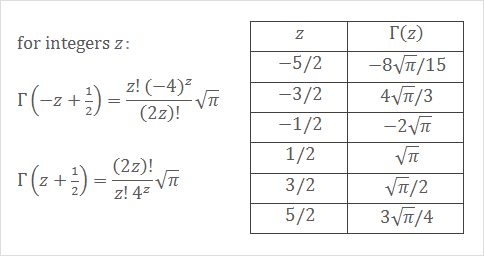One can also express Γ(1/4) and Γ(1/3) in terms of π and Arithmetic-Geometric Means: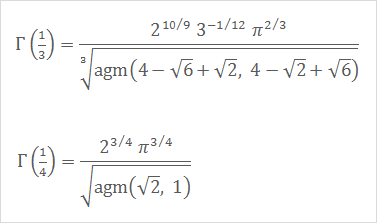## The Log Gamma Function

The log gamma function is defined as Ln(Γ(z)). Since the gamma function grows quickly, it is sometimes easier to make calculations with the natural logarithm of the gamma function.

## Related Functions

The Beta Function B(x,y) is defined as

B(x,y) = Γ(x)Γ(y)/Γ(x+y).

The Digamma Function ψ(z) is defined as

ψ(z) = Γ'(z)/Γ(z) = d/dz[Ln(Γ(z))].